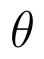Select Page

# MCQ Relations and Functions CBSE Maths 12 Science Answers in English

MCQ Relations and Functions CBSE Maths 12 Science Answers in English to enable students to get Answers in a narrative video format for the specific question.

Expert Teacher provides MCQ Relations and Functions CBSE Maths 12 Science Answers through Video Answers in English language. This video solution will be useful for students to understand how to write an answer in exam in order to score more marks. This teacher uses a narrative style for a question from Relations and Functions not only to explain the proper method of answering question, but deriving right answer too.

Please find the question below and view the Answer in a narrative video format.

Question:

## Similar Questions from CBSE, 12th Science, Maths, Relations and Functions

Question 1 : Let * be the binary operation on N given by a * b = LCM of a and b. Find the identity of * in N? (View Answer Video)

Question 2 :be  defined as f(x) =(View Answer Video)

Question 3 :  If f(x) = ax + b and g(x) = cx + d, then f[g(x)] – g[f(x)] is equivalent to, (View Answer Video)

Question 4 : Functionsare defined respectively, by, find. (View Answer Video)

Question 5 : Functionsare defined respectively, by, find(View Answer Video)

### Matrices

Question 1 : If,find the value of x. (View Answer Video)

Question 2 : LetFind A - B. (View Answer Video)

Question 3 : Compute:. (View Answer Video)

Question 4 : Find the value of y from the equation:(View Answer Video)

Question 5 : Using elementary transformation, find the inverse of the matrix. (View Answer Video)

### Three Dimensional Geometry

Question 1 : If a line marks anglesandwith x, y and z-axis respectively, whereis acute, then find. (View Answer Video)

Question 2 : Find the vector equation of a plane which is at a distance of 5 units from the origin and its normal vector is(View Answer Video)

Question 3 : If a line has the direction ratios -18, 12, -4, then what are its direction cosines? (View Answer Video)

Question 4 : Find the vector and cartesian equations of the plane passing through the point (-1, 3, 2) and perpendicular to each of the planes x + 2y +3z = 5 and

Question 5 : Find the equation of the plane passing through the line of intersection of the planes x + y + z = 1 and 2x + 3y + 4z = 5 which is perpendicular to the plane x - y + z = 0. Also, find the distance of the plane obtained above from the origin. (View Answer Video)

### Differential Equations

Question 3 : Write the degree of the differential equation :(View Answer Video)
Question 4 : Write the differential equation representing the curvewhere a is an arbitrary constant. (View Answer Video)